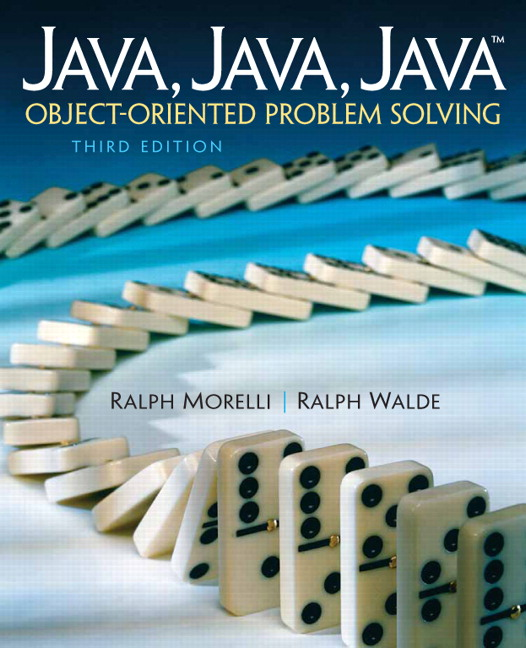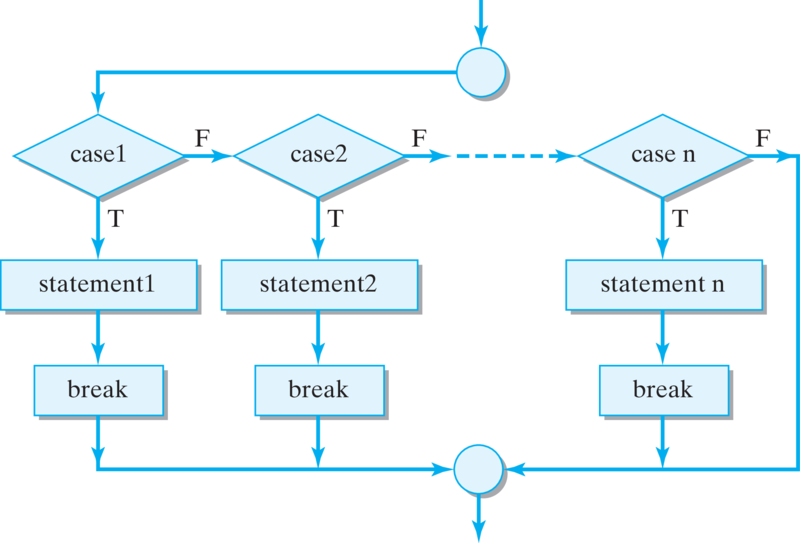# Java, Java, Java: Object-Oriented Problem Solving, 2022E

## Section6.10The switch Multiway Selection Structure

### Subsection6.10.1Switch

Another selection structure to add to our repertoire is the switch/break structure. It is meant to provide a shorthand way of coding the following type of multiway selection structure:
if (integralVar == integralValue1)
// some statements
else if (integralVar == integralValue2)
// some statements
else if (integralVar == integralValue3)
// some statements
else // some statements

Note that each of the conditions in this case involves the equality of an integral variable and an integral value. This type of structure occurs so frequently in programs that most languages contain statements specially designed to handle it. In Java, we use a combination of the switch and break statements to implement multiway selection.
The switch is designed to select one of several actions depending on the value of some integral expression:
switch (integralExpression)
{  case integralValue1:
// some statements
case integralValue2:
// some statements
case integralValue3:
// some statements
default:
some statements
}

The integralExpression must evaluate to a primitive integral value of type byte, short, int, char, or boolean. It may not be a long, float, double, or a class type. The integralValues must be literals or final variables. They serve as labels in the one or more case clauses that make up the switch statement body. The default clause is optional, but it is a good idea to include it.
A switch statement is executed according to the following rules:
1. The integralExpression is evaluated.
2. Control passes to the statements following the case label whose value equals the integralExpression or, if no cases apply, to the default clause.
3. Beginning at the selected label or at the default, all of the statements up to the end of the switch are executed. \end{NL}
Consider the following example:
int m = 2;
switch (m)
{
case 1:
System.out.print(" m = 1");
case 2:
System.out.print(" m = 2");
case 3:
System.out.print(" m = 3");
default:
System.out.print(" default case");
}

In this case, because m equals 2, the following output would be produced:
m = 2 m = 3 default case

Obviously, this output does not match the following if-else multiway selection structure, which would output, simply, m = 2:
int m = 2;
if (m == 1)
System.out.print(" m = 1");
else if (m == 2)
System.out.print(" m = 2");
else if (m == 3)
System.out.print(" m = 3");
else
System.out.print(" default case");

The reason for this disparity is that the switch executes all statements following the label that matches the value of the integralExpression (see again Rule 3 above).
In order to use the switch as a multiway selection, you must force it to break out of the case clause after executing that clause’s statements:
int m = 2;
switch (m)
{  case 1:
System.out.print(" m = 1");
break;
case 2:
System.out.print(" m = 2");
break;
case 3:
System.out.print(" m = 3");
break;
default:
System.out.print(" default case");
}

In this example, the break statement causes control to pass to the end of the switch, with the effect being that one and only one case will be executed within the switch. Thus, the output of this code segment will be simply m = 2 , matching exactly the behavior of the multiway if-else selection structure (Figure 6.10.1).Figure 6.10.1. Flowchart of the multiway switch structure. Note that because of the break statement, one and only one case is executed.

#### ExercisesSelf-Study Exercises

##### 1.Switch Bug 1.
Identify and fix the errors in the following switch structure.
##### 2.Switch Bug 2.
Identify and fix the errors in the following switch structure.
##### 3.Switch Bug 3.
Identify and fix the errors in the following switch structure.
##### 4.Ice Cream Flavors.
Write a switch statement that checks an integer variable flavor and prints out the name of the ice cream flavor (where 0 is vanilla, 1 is chocolate, and 2 is strawberry) or prints “Error” in the default case. Test by changing the flavor variable’s value. Then, modify your solution to use constants (final variables) to represent the ice cream flavors.